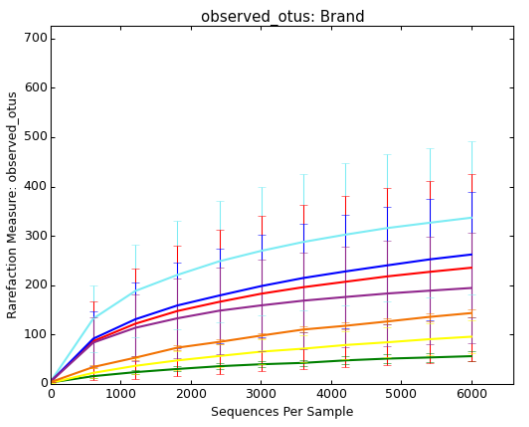# Alpha Diversity Boxplot

Hello QIIME developers,

I wanted to plot the alpha rarefaction of my data, but the fonts are too small and the colours are not what I want. So, I remembered using QIIME1, and the plots are much clearer, so I used QIIME1.

Then, I realized that the size of boxplots/error bars are very different. Did I do something wrong here?

Here is my attempt in QIIME2 (there are 60 samples, grouped by one particular sample metadata, 'Brand'):

And here is my attempt in QIIME1:You can also see that there are three lines above 200 observed otus in QIIME2 figure, but two lines in QIIME1 figure. The two most diverse 'Brand's (two highest lines) in QIIME2 figure are close to each other, which is not the case in QIIME1 figure.

I also tried making my own line plots of individual brands (not as decent as figures produced in QIIME2, unfortunately). I collapsed all values of the same brand and the figure appeared similar to QIIME1 figures:

Thank you and have a nice day.

Hey there @ZarulSaurus!

QIIME 2 is plotting the median values for the lines, and the boxplots are showing the quartiles (25th and 75th percentiles) on the box, and +/- 1.5 IQR for the whiskers.

QIIME 1 is plotting the mean values for the lines, and the error bars are the standard deviation (unless you overrode those parameters).

Can you provide some details about how you generated your plot (the third plot, homemade)? Specifically, did you plot the mean or the median? If you plotted the mean, would you be able to regenerate the plot with the median values and share that here?

Hello, thank you very much for your response. Fortunately, I was able to reproduce the QIIME2 lines using median values:

However, when I added boxplots, it doesnt look like the ones in QIIME2. Aside from the outliers, the boxes are mostly overlapping, which is not the case for visualizations in QIIME2:

This is how the plot appears when samples are separated by brands:

I plotted them using matplotlib. The default behavior of matplotlib.pyplot.boxplot is that the "box extends from the lower to upper quartile values of the data", and the whiskers are Q3 + 1.5(IQR) and Q1 - 1.5(IQR), just like your description of QIIME2 alpha rarefaction plots.

Here is the observed_otus.csv, in case if you want to have a look at the data:

observed_otus.csv (37.5 KB)

2 Likes

Sorry @ZarulSaurus - I misspoke:

``````                  The box plots in the upper figure represent the distribution
of the selected alpha diversity metric for each group of
samples at each even sampling depth. The lower and upper
whiskers of the box plot are the 9th and 91st percentiles of
the distribution (respectively), while the lower and upper
extents of the box are the 25th and 75th percentiles of the
distribution (respectively). The horizontal bar through the
middle of the box is the median  of the dstribution
(i.e., the 50th percentile). Outlier points of these
distributions are not shown.
``````

Source

1 Like

This topic was automatically closed 31 days after the last reply. New replies are no longer allowed.